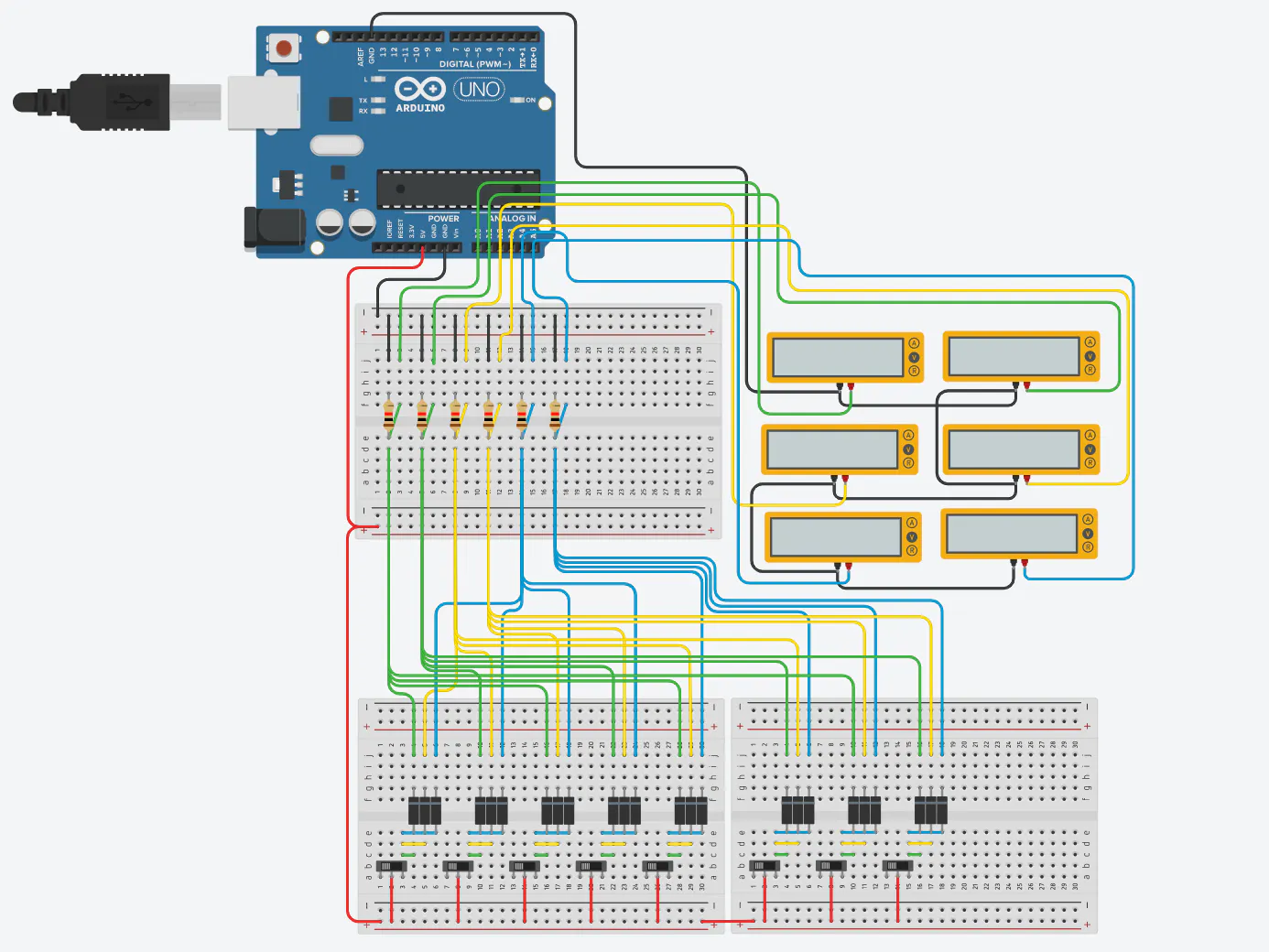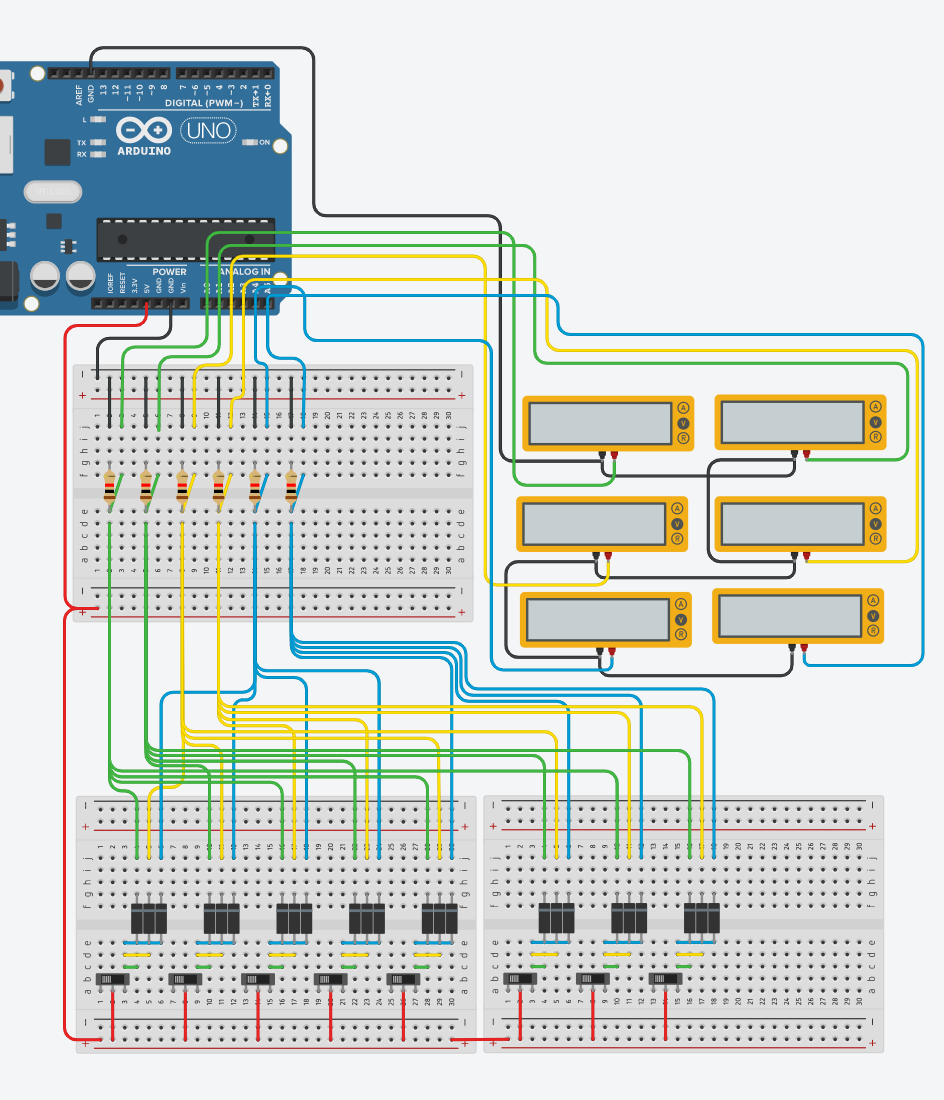Project in progress# Maximize Inputs

• 1,993 views
• 8 respects

## Components and supplies

So like many of us we always run out of inputs and multiplexing is fine but why not work the problem another way. So I thought up this way to get 125 inputs into a mega 2560 with out anything to special.

This is a proof of concept using 6 inputs to get 8 buttons onto a Uno and then simulate the environment in 123D Circuits.

There are some conditions that limit the ability of the circuit. These conditions I believe are mostly rooted in vector mathematics or it has to do with the way I set it up. Although if you only use one button at a time it works great.

Setting up this involves separating signal into three parts and sending that signal into its designated X, Y, Z component. You have to use the diodes to prevent any signal from wondering around to other buttons based on common end points.

I used different colored wire to signify the X, Y, Z location. Green for X, Yellow for Y, and Blue for Z. The list of combinations can be found in the code.

Once all the signals are going to there proper locations we use the resistors to pull the signals down to ground to keep our button pushes clean. I used multi meters to check my combinations as a physical debugging tool.

I am still working the problem with the end goal of getting all 125 on with 100% accuracy with out any false positives.

## Code

##### 2x2x2 Cubed InputsArduino
Gives you the ability to get more buttons than inputs
```/*
Created by:

Amos Parmenter
July 20th 2019

The code is setup with the idea that you can
fit more buttons onto the Arduino that inputs allow.
The code uses the idea of assigning vector
identity to a node that will be unique when compared to other nodes.

This version is setup as 2x2x2 cube and can fit 8 buttons
into 6 analog slots. It is scalable in cubed
form (3x3x3.(mega), 4x4x4.(mega) , 5x5x5.(mega maxed inputs)).
The 5x5x5 uses 15 analog pins but in return gives you 125 buttons.

There are mathematical complications to this code that
I haven’t fully worked out.To be sure you don’t get false
positives for buttons you can only turn on one button at a time.
In most cases you can have two buttons on at once but not
every two combinations are allowed.
Any three combinations will always give you false positives.
The simulations I wired in 123D Circuits with the 2x2x2
cubed wiring and is running this version of code.

*/

const int x0 = 0; // input pins
const int x1 = 1; // input pins
const int y0 = 2; // input pins
const int y1 = 3; // input pins
const int z0 = 4; // input pins
const int z1 = 5; // input pins

int x_0; // nodes
int x_1; // nodes
int y_0; // nodes
int y_1; // nodes
int z_0; // nodes
int z_1; // nodes

bool btn0 = false;
bool btn1 = false;
bool btn2 = false;
bool btn3 = false;
bool btn4 = false;
bool btn5 = false;
bool btn6 = false;
bool btn7 = false;

bool vectorX = false;
bool vectorY = false;
bool vectorZ = false;

bool btnBoolMatrix[] = {btn0, btn1, btn2, btn3,
btn4, btn5, btn6, btn7
};

int analogList = {
{x0, y0, z0},
{x1, y0, z0},
{x0, y1, z0},
{x1, y1, z0},
//============
{x0, y0, z1},
{x1, y0, z1},
{x0, y1, z1},
{x1, y1, z1}
};

int btnMatrix = {
{x_0, y_0, z_0},
{x_1, y_0, z_0},
{x_0, y_1, z_0},
{x_1, y_1, z_0},
//============
{x_0, y_0, z_1},
{x_1, y_0, z_1},
{x_0, y_1, z_1},
{x_1, y_1, z_1}
};

bool vectorXYZ  {vectorX, vectorY, vectorZ};

void setup()
{
Serial.begin(9600);

pinMode(x0, INPUT);
pinMode(x1, INPUT);
pinMode(y0, INPUT);
pinMode(y1, INPUT);
pinMode(z0, INPUT);
pinMode(z1, INPUT);

}

void loop() {

/* This section allows you to check
the active button by entering the number
1 into the Serail monitor
*/

while (Serial.available() < 0);
int options = Serial.read() - '0';
if (options == 1) {

checkBtnList();
}

}

void checkBtnList() {

for (int i = 0; i < 8; i++) {

for (int i = 0; i < 3; i++) {
vectorXYZ[i] = false;
}

for (int j = 0; j < 3; j++) {

if (btnMatrix[i][j] > 250 ) {
vectorXYZ[j] = true;
} else {
vectorXYZ[j] = false;
}

if (j == 2) {
if (vectorXYZ == true && vectorXYZ == true && vectorXYZ == true) {
btnBoolMatrix[i] = true;
} else {
btnBoolMatrix[i] = false;
}
}

}
}

for (int s = 0; s < 7; s++) {
Serial.print(btnBoolMatrix[s]);
Serial.print(" ");
}
Serial.println(btnBoolMatrix);

}
```

## Schematics

the complete 2x2x2 circuit wiringJuly 29, 2019

#### Members who respect this project

See similar projects
you might like

#### Arduino Serial Plotter & Capacitors

Project in progress by Koiotti

• 2,210 views
• 5 respects

#### Arduino Bluetooth Robot for Android Device

Project showcase by aip06

• 4,500 views
• 19 respects

• 16,627 views
• 43 respects

#### How Easy Is It to Use a Thermistor?!

Project tutorial by Marcazzan_M

• 14,216 views
• 14 respects

#### Healthcare Skin Pressure Detector

Project tutorial by David Escobar

• 9,457 views